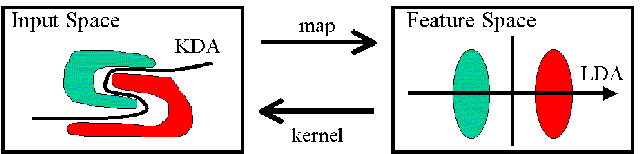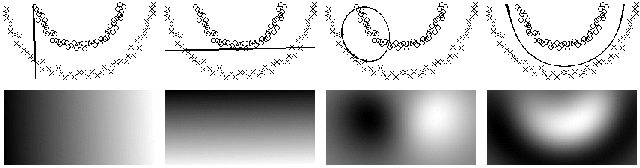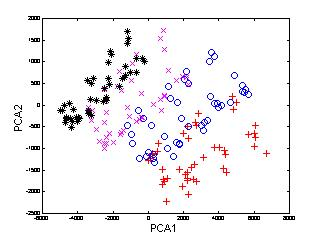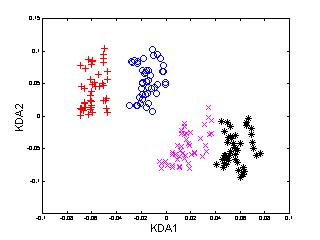Kernel Discriminant Analysis

Yongmin Li, Shaogang Gong and Heather Liddell
Department of Computer Science
Queen Mary, University of London

1. Introduction

For most pattern recognition problems, selecting an appropriate representation to extract the most significant features is crucially important. Principal Component Analysis (PCA) [8,10] has been widely adopted to extract abstract features and to reduce dimensionality in many pattern recognition problems. But the features extracted by PCA are actually "global" features for all pattern classes, thus they are not necessarily much representative for discriminating one class from others. Linear Discriminant Analysis (LDA) [2,3,9], which seeks to find a linear transformation by maximising the between-class variance and minimising the within-class variance, has proved to be a suitable technique for discriminating different pattern classes. However, both the PCA and LDA are linear techniques which may be less efficient when severe non-linearity is involved. To extract the non-linear principal components, Kernel PCA (KPCA)  was developed using the popular kernel technique [11,12]. However, similar to the linear PCA, KPCA captures the overall variance of all patterns which are not necessary significant for discriminant purpose.

To extract the nonlinear discriminant features, Kernel Discriminant Analysis (KDA) [6,1,4], a nonlinear discriminating method based on kernel techniques [11,12], was developed.

2. Kernel Discriminant Analysis

The principle of KDA can be illustrated in Figure 1. Owing to the severe non-linearity, it is difficult to directly compute the discriminating features between the two classes of patterns in the original input space (left). By defining a non-linear mapping from the input space to a high-dimensional feature space (right), we (expect to) obtain a linearly separable distribution in the feature space. Then LDA, the linear technique, can be performed in the feature space to extract the most significant discriminating features. However, the computation may be problematic or even impossible in the feature space owing to the high dimensionality. By introducing a kernel function which corresponds to the non-linear mapping, all the computation can conveniently be carried out in the input space. The problem can be finally solved as an eigen-decomposition problem like PCA, LDA and KPCA.3. A Toy Problem

We use a toy problem to illustrate the characteristics of KDA as shown in Figure 2. Two classes of patterns denoted by circles and crosses respectively have a significant non-linear distribution. To make the results comparable among different representations (PCA, LDA, KPCA and KDA), we try to separate them with a one dimensional feature, i.e. the most significant mode of PCA, LDA, KPCA or KDA. The first row shows the patterns and the discriminating boundaries computed by each of the four different methods. The second row illustrates the intensity of the one-dimensional features given by PCA, LDA, KPCA and KDA on the region covered by the training patterns.4. Representing Multi-View Faces Using KDA

Face images with large pose variation demonstrate significant nonlinearity, which makes face recognition a challenging problem. To address this problem, we apply KDA to represent multi-view face patterns.

In the following example (Figure 3), 540 multi-view face patterns from 12 subjects, 45 of each, were used to train KDA. For the sake of clarity, only the patterns of first four face classes are shown here. To compare the performance of KDA, we also applied PCA, LDA and KPCA to the same set of face patterns.It is noted that

1. the pattern distributions using PCA and KPCA are not satisfactorily separable, or more precisely, the variation from different subjects is even overshadowed by that of other sources, since these two techniques are not designed for discriminating purpose;
2. LDA performs better than PCA and KPCA, but not as good as KDA;
3. KDA provides the best discriminating performance among the four methods.

Yongmin Li 2001-10-14